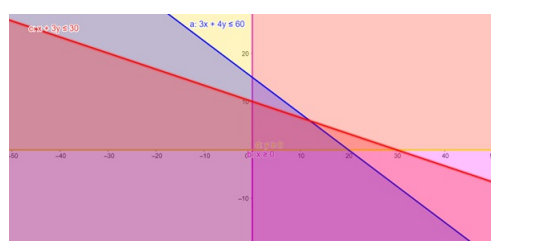# Solve the given inequalities

Question:

Solve the given inequalities $3 x+4 y \leq 60, x+3 y \leq 30, x \geq 0, y \geq 0$ graphically in two - dimensional plane.

Solution:

The graphical representation of $3 x+4 y \leq 60, x+3 y \leq 30, x \geq 0, y \geq 0$ is given by common region in the figure below.

$3 x+4 y \leq 60 \ldots \ldots$ (1)

$x+3 y \leq 30 \ldots \ldots$ (2)

$x \geq 0 \ldots \ldots$ (3)

$y \geq 0 \ldots \ldots$ (4)

Inequality (1) represents the region below line $3 x+4 y=60$ (including the line $3 x+4 y=60$ ).

Inequality (2) represents the region below line $x+3 y=30$ (including the line $x+3 y=30$ ).

Inequality (3) represents the region in front of line $x=0$ (including the line $x=0$ ).

Inequality (4) represents the region above line $y=0$ (including the line $y=0$ ).

Therefore,every point in the common shaded region including the points on the respective lines represents the solution for the given inequalities.

This can be represented as follows,